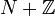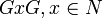## Definition

Suppose$N$ is a radical ring and$G$ is its adjoint group, i.e.,$G = 1 + N$.

The supercharacter theory of$G$ is a supercharacter theory defined as follows.

### Superconjugacy classes

Note that$G$ is a subgroup of the unitization$N + \mathbb{Z}$, hence acts on$N + \mathbb{Z}$ by both left and right multiplication. The ideal$N$ is invariant under both actions, so we get a left and a right$G$-action on$N$ that commute with each other. Consider the double orbits of$N$ under these actions, i.e., sets of the form$GxG, x \in N$. The corresponding sets$1 + GxG$, which are subsets of$G$, are the superconjugacy classes.

Note that the conjugation action of$G$ on$N$ corresponds to its conjugation action on itself, and the orbits for this conjugation action are contained inside the double orbits, so the superconjugacy classes are unions of conjugacy classes.

Note also that even if we treat$N$ as a$R$-algebra for a commutative unital ring$R$ other than$\mathbb{Z}$ and consider the corresponding unitization, the superconjugacy class structure is the same.